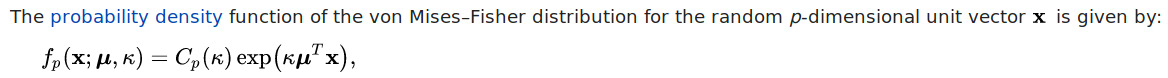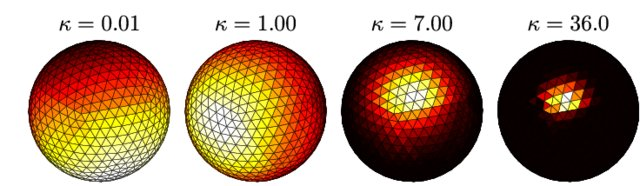# Highlights

• Deep learning tractography method
• Predicts the parameters for a unimodal distribution on the sphere (Fisher von Mises)
• Uncertainty quantification
• Maximum-entropy regularization as loss function instead of NLL to enforce non-zero uncertainty and robustness to noise

# Method

Model: Simple Multi-Layer perceptron

Input:

• Block of 3x3x3 voxels of DWI data fitted with spherical harmonics of order 4 (15 values per voxel = 405 features)
• 4 last directions (3 values per direction = 12 features)

Prediction: Parameters of a Fisher von Mises distribution:(Taken from Wikipedia)(Taken from )

The Fisher von Mises distribution is analogous to a Gaussian on the Sphere. It has a mean parameter $$\mu$$ and a concentration parameter $$\kappa$$.

Loss function: Total free energy given the predicted distribution parameters (and a temperature hyper-parameter $$T$$), which can be calculated analytically for the Fisher von Mises distribution

The model is optimized using SGD and gradient clipping.

# Experiments

• Training: 1 HCP subject, tracked using a probabilistic algorithm.

• Testing: Tractometer tool (synthetic signal, 25 GT bundles to reconstruct)

• Output metrics: Valid Bundles (VB), Invalid Bundles (IB), Valid Connections (VC), Overlap (OL), Overreach (OR), F1-score (using overlap + overreach)

## Results

The Entrack model achieves scores comparable to the other works, indicating its usefulness as predictive model for fiber tracking, in addition to its benefits of uncertainty quantification, and outlier detection.

• See figure 6: The model is also trained on the tractometer data and used to color the training streamlines according to the total probability ((a) and (b) or local uncertainty ((c) and (d)).

: Plis, S., McCracken, S., Lane, T., & Calhoun, V. (2011, June). Directional statistics on permutations. In Proceedings of the Fourteenth International Conference on Artificial Intelligence and Statistics (pp. 600-608).